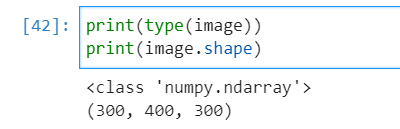# How to convert 3D numpy array to vtk and save the .vtk file?

Hi all,I am new here.Sorry for my little knowledge about VTK.
I have a scalar volume (3D numpy array)I want to add some information describing the image and then save it as a .vtk file as follows:I searched some codes below:

``````import vtk.util.numpy_support as numpy_support

def numpyToVTK(data):
data_type = vtk.VTK_FLOAT
flat_data_array = data.transpose(2,1,0).flatten()
vtk_data = numpy_support.numpy_to_vtk(num_array=flat_data_array, deep=True, array_type=data_type)
shape = data.shape
img = vtk.vtkImageData()
img.GetPointData().SetScalars(vtk_data)
img.SetDimensions(shape, shape, shape)
return img
``````

I am not sure the code is right or not and I want to check the dimension of the array step by step,also how to save the file on hard-disk?
I will appreciate it if someone can give me some illustration! Thank you for your help in advance!